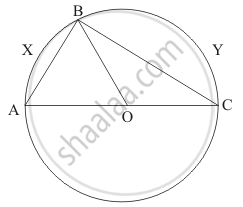Advertisement Remove all ads

# If the Given Figure, Aoc is a Diameter of the Circle and Arc Axb = 1 2 Arc Byc. Find ∠Boc. - Mathematics

Answer in Brief

If the given figure, AOC is a diameter of the circle and arc AXB = $\frac{1}{2}$ arc BYC. Find ∠BOC.Advertisement Remove all ads

#### Solution

We need to find  angle BOC$\text{ arc } AXB = \frac{1}{2}\text{ arc} BYC,$

$\angle AOB = \frac{1}{2}\angle BOC$

$\text{ Also } \angle AOB + \angle BOC = 180°$

$\text{ Therefore, } \frac{1}{2}\angle BOC + \angle BOC = 180°$

$\Rightarrow \angle BOC = \frac{2}{3} \times 180°= 120°$

Is there an error in this question or solution?
Advertisement Remove all ads

#### APPEARS IN

RD Sharma Mathematics for Class 9
Chapter 15 Circles
Q 9 | Page 109
Advertisement Remove all ads

#### Video TutorialsVIEW ALL 

Advertisement Remove all ads
Share
Notifications

View all notifications

Forgot password?
Course